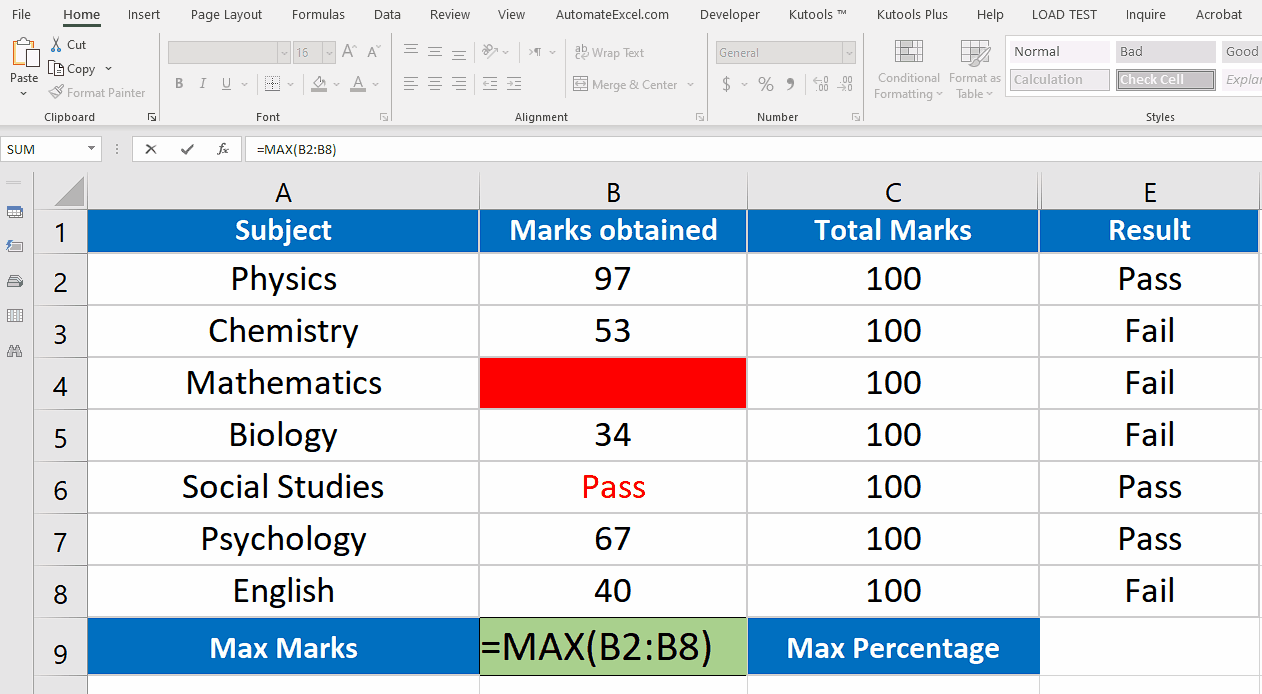# How to use Max function in Excel

Using the MAX function we can process a maximum of 255 values at a time. The values can be constants or cell references. It will work only on numeric data and will ignore all the blanks, boolean values (TRUE, FALSE) or text values as well. Let’s take a look at the data of a student above picture and find out what is the highest score he got out of all subject scores.The MAX function is a very simple yet useful function in Excel which can be found under the statistical functions category.

If we are dealing with a small data set then it seems to be very easy to locate the maximum value inside the data by just looking at the data. However, when you have many rows of data and that too consisting of repeating values then it could become very difficult to locate the maximum value of such a big data.

That’s where the Max() functions comes into play and can help us finding the maximum value out of a big data set with just one simple formula i.e.

=MAX(value1, value2,…)

### Step 1 – Write the formula in an appropriate cell– Write the formula in Cell B9 to obtain the maximum value of marks obtained by the student. Notice that the data set contains a blank cell filled with red and a text value

Pass also marked with red font color.

### Step 2 – Implement the formula and get results– Write the formula in Cell B9 to obtain the maximum value of marks obtained by the student.

– We’ll see that max function will get us the maximum marks in the data set ignoring blank and text values.